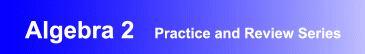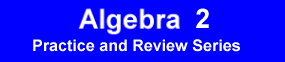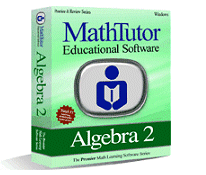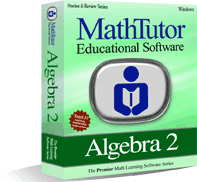Award-Winning

# Algebra 2 Practice and Review SoftwareDESCRIPTION
ALGEBRA 2 is a software package designed to help students develop problem solving skills in intermediate algebra through carefully crafted drill and practice exercises.
ALGEBRA 2 also allows students to reinforce their understanding of the concepts of intermediate algebra through problem solving. It is research based and designed by math curriculum specialists. The program provides carefully designed practice and drill exercises tailored to each student's ability.
Problem solving exercises progress gradually from the simpler to the more challenging. Problems are randomized and based on students' skill levels.
The program helps students identify what a problem is asking and what is required to carry out a solution. Step by step explanations help students learn how to carry out the process.
Feedback and recordkeeping provide encouragement and help motivate students.
• Curriculum-based advanced algebra drill and practice software developed by math educators
• Dynamic, interactive intermediate algebra problem solving exercises that are ideal for self-study, and as a supplement to classroom instruction
• Demonstrated improvement in problem solving skills as well as students' understanding of conceptsTOPICS COVERED
Systems of Equations and Determinants
• Definitions and Concepts
• Systems of Two Eqns: Equivalent Eqns
• Systems of Two Eqns: Solutions
• Systems of Three Eqns: Equiv. Eqns
• Systems of Three Eqns: Solutions
• Translating Statements to Equations
• Determinants: 2 x 2
• Determinants: 3 x 3
• Systems of Two Eqns: Word Probs
• Systems of Three Eqns: Word Probs
• Word Problems: A Grass Mixture
• Word Problems: A Money Collection
Polynomials and Rational Functions
• Definitions and Concepts
• Polynomials: Elementary
• Proportions: Direct and Indirect
• Polynomials: Roots
• Polynomials: Qualitative
• Complex Numbers
• Absolute Value
• Word Problems: Filling a Can
• D = RT
• Word Problems: Animals on a Farm
• Word Problems: Averages
and Conics

• Definitions and Concepts
• Logarithms
• Equations: Parameters
• Exponents I
• Exponents II
• Functions: Comparisons
• Curves in the Plane
• Conics: Analytic
• Conics: Properties
• Conics: Graphs